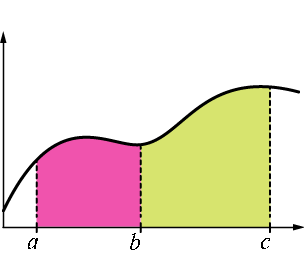## Properties of the Definite Integral

Recall that the definition of the definite integral (given again below) has a summation at its heart.

$$\int_a^b f(x)\,dx = \lim_{||\Delta|| \rightarrow 0} \sum_{i=1}^n f(x_i^*) \Delta_i$$

Whether through playing around with this summation or through other means, we can develop several important properties of the definite integral. We consider several of these below, in turn.

$\displaystyle{\int_a^b f(x)\,dx = -\int_b^a f(x)\,dx}$

Suppose, as we have in the past, that the partition associated with the Riemann sum is given by $a = x_0 \lt x_1 \lt x_2 \lt \cdots \lt x_n = b$.

Now recall that $\Delta_i = x_i - x_{i-1}$, which changes sign when the limits of integration are switched from $\displaystyle{\int_a^b}$ to $\displaystyle{\int_b^a}$.

This introduces a $(-1)$ as a factor on each term of the Riemann sum, which can then be pulled outside of the summation, and then the limit.

$\displaystyle{\int_a^a f(x)\,dx = 0}$

Of course, if the interval in question is $[a,a]$, any $\Delta_i$ present in the Riemann sum would have to be zero -- which in turn makes the sum and its limit zero as well.

$\displaystyle{\int_a^b (f(x) \pm g(x))\,dx = \int_a^b f(x)\,dx \pm \int_a^b g(x)\,dx}$

Suppose $F$ is an antiderivative of $f$, and $G$ is an antiderivative of $g$. Then $F \pm G$ must be an antiderivative of $f \pm g$. This means that

$$\begin{array}{rcl} \int_a^b (f(x) \pm g(x))\,dx &=& (F(x) \pm G(x))\,\bigg\rvert_a^b\\\\ &=& (F(b) \pm G(b)) - (F(a) \pm G(a))\\\\ &=& (F(b)-F(a)) \pm (G(b)-G(a))\\\\ &=& \displaystyle{\int_a^b f(x)\,dx \pm \int_a^b g(x)\,dx} \end{array}$$

If $f(x) \ge 0$ where $a \le x \le b$, then $\displaystyle{\int_a^b f(x)\,dx \ge 0}$

Knowing $f(x) \ge 0$ tells us each $f(x_i^*) \ge 0$. Further, since $a \le b$ we also know $\Delta_i \ge 0$. Thus every term in the summation below that defines the definite integral is non-negative, making the sum (and consequently the value of the limit) non-negative.

$$\int_a^b f(x)\,dx = \lim_{||\Delta|| \rightarrow 0} \sum_{i=1}^n f(x_i^*) \Delta_i$$

If $f(x) \ge g(x)$ where $a \le x \le b$, then $\displaystyle{\int_a^b f(x)\,dx \ge \int_a^b g(x)\,dx}$

Let $h = f - g$, and then note that $h(x) \ge 0$ for all $a \le x \le b$. The previous result then applies and tells us $\int_a^b h(x)\,dx \ge0$. Equivalently, $\int_a^b (f(x)-g(x))\,dx = \int_a^b f(x)\,dx - \int_a^b g(x) \ge 0$.

So,

$$\int_a^b f(x)\,dx \ge \int_a^b g(x)\,dx$$

$\displaystyle{\int_a^b f(x)\,dx + \int_b^c f(x)\,dx = \int_a^c f(x)\,dx}$

We can prove this in a rigorous way for any values $a$, $b$, and $c$ and integrable function $f$, but the following argument might be more illuminating. Suppose $a \lt b \lt c$ and $f(x)$ is non-negative, as suggested by the image below.In this context, note that $\int_a^b f(x)\,dx$ gives the area under the curve from $a$ to $b$ (shown in pink), while $\int_b^c f(x)\,dx$ gives the area under the curve from $b$ to $c$ (shown in light green). Clearly, the sum of these two is the area under the from $a$ to $c$, given by $\int_a^c f(x)\,dx$.